# How to Use Different Data Visualizations in the Grafana Dashboard

In this post, we will see how to use different visualizations, like the simple graph, pie chart, or world map panel in the Grafana dashboard. We'll do this by writing queries in the Influx query language and fetching data from the InfluxDB database which is a time-series database.

• a. Data Visualization
• b. Installing Plugins Docker Grafana
• c. Writing to the InfluxDB2.0

## LINE CHARTS:

1. As we can see, we are showing the records for 2 different blocks, i.e, DS_Id = 0 and DS_Id = 1.The timestamp is the same for both the blocks, 1598918400, 1598918402, 15989The date for the above can be obtained from the link: https://www.epochconverter.com/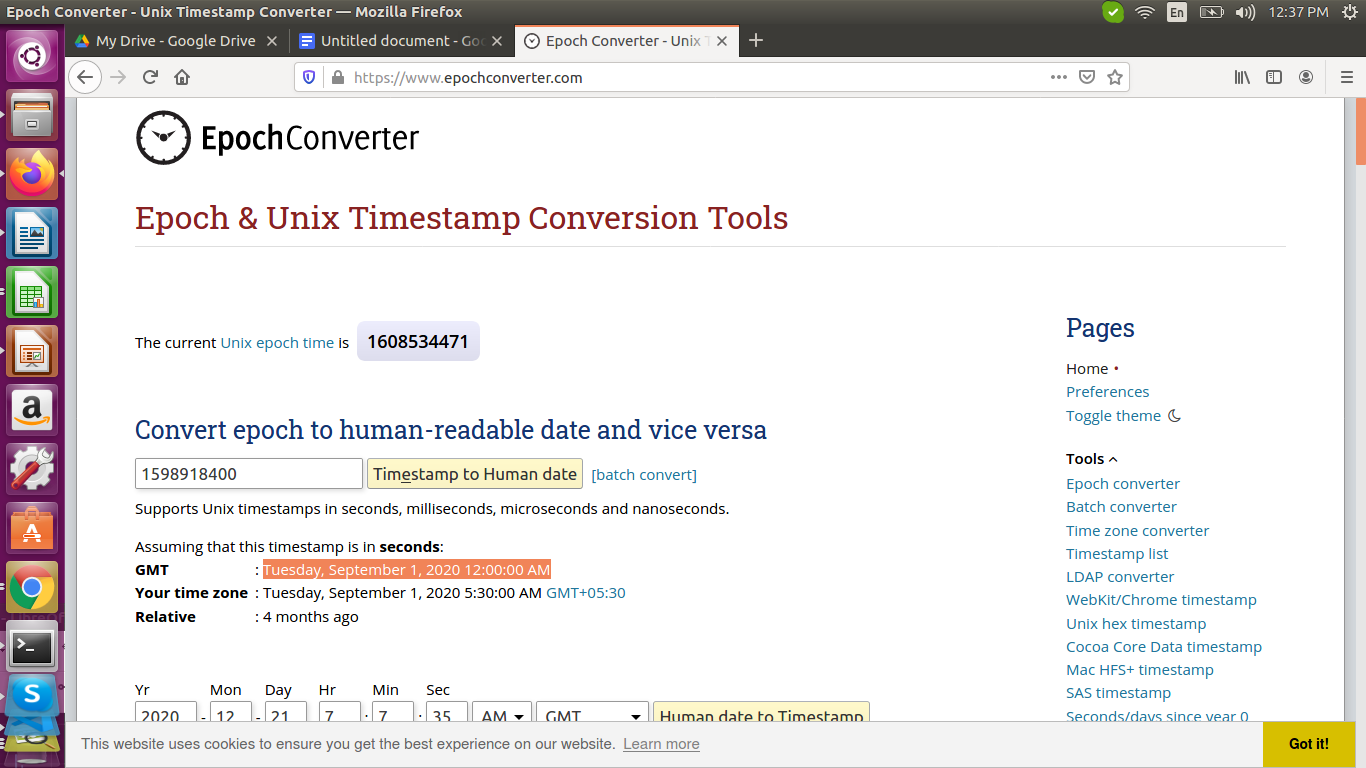TIMESTAMP

DS_Id

POWER_A

POWER_B

POWER_C

1598918400

0

1403.421

712.372

1680.471

1598918402

0

1423.817

731.249

1680.658

1598918403

0

1444.172

749.339

1700.859

1598918404

0

1774.402

1106.427

2041.954

1598918405

0

1774.402

1106.427

2041.954

TIMESTAMP

DS_Id

POWER_A

POWER_B

POWER_C

1598918400

1

821.847

574.748

1203.807

1598918402

1

823.367

574.315

1203.795

1598918403

1

819.939

574.261

1203.647

1598918404

1

819.939

574.261

1203.647

Our requirement is to get the aggregated power for POWER_A, POWER_B, POWER_C fields.

For example, for the timestamp 1598918400:

We have values for POWER_A as 1403.421W and 821.847W, sum as 2225.268W. Likewise, we have to calculate for all the time-series values(1598918402, 1598918403, 1598918404, …) for POWER_A, POWER_B and POWER_C also.

This computation is done in Grafana. We are implementing this using the SQL syntax like queries as shown below:

We are going to compute the aggregated power for the field POWER_A now.

All the queries were constructed and executed successfully by Narendra Reddy Mallidi, SQL Developer.

We have our first query below:

SELECT POWER_A as AA FROM "hdb2" WHERE "DS_ID" = '0'


Here, POWER_A is the variable where are going to fetch from the table(table is called measurement in InfluxDB queries) named "hdb2".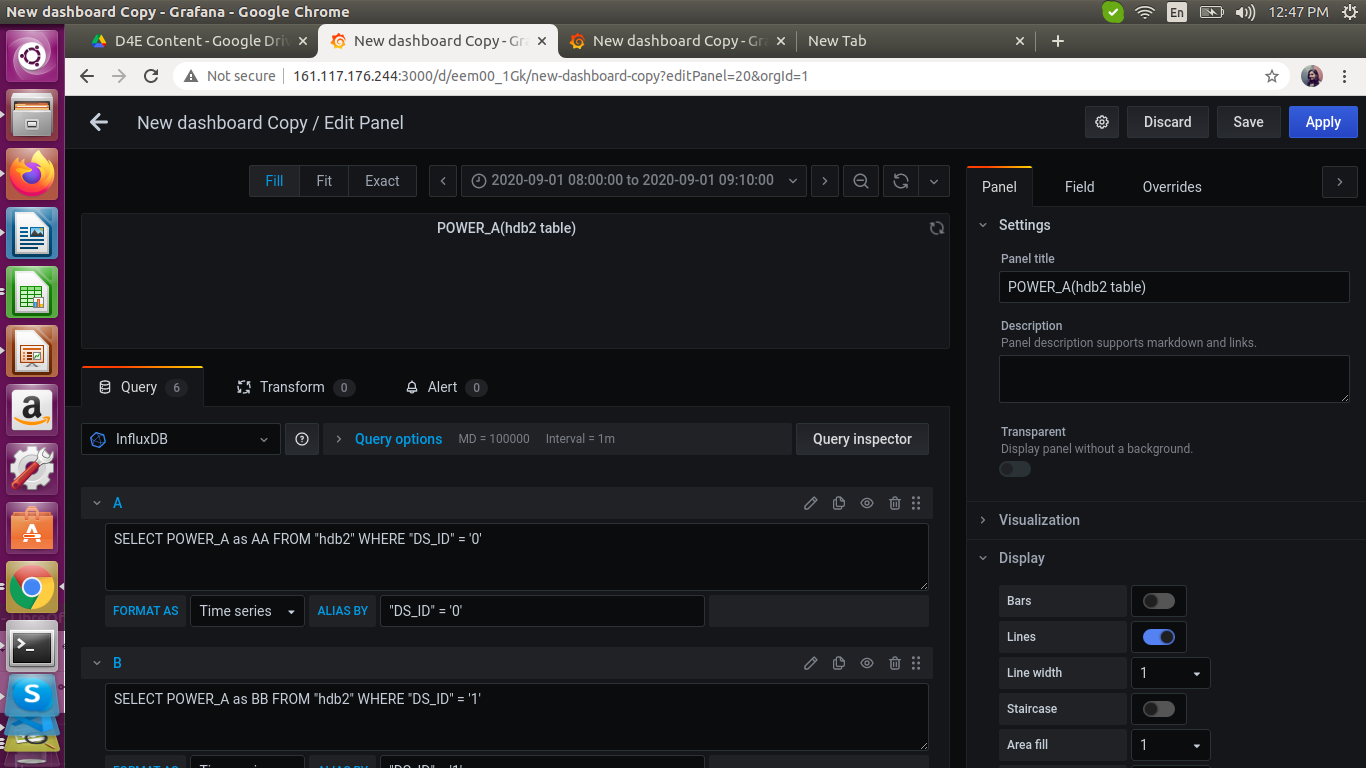The same thing is followed for other 4 blocks also(DS_ID =’1’, DS_ID=’2’, DS_ID=’3’, DS_ID=’4’)

SELECT POWER_A as BB FROM "hdb2" WHERE "DS_ID" = '1'
SELECT POWER_A as CC FROM "hdb2" WHERE "DS_ID" = '2'
SELECT POWER_A as DD FROM "hdb2" WHERE "DS_ID" = '3'
SELECT POWER_A as EE FROM "hdb2" WHERE "DS_ID" = '4'


Now, we compute the aggregated power for POWER_A with the below query:

SELECT sum(ds1) + sum(ds0) + sum(ds2) + sum(ds3) + sum(ds4) AS Total
FROM
(
SELECT POWER_A as ds0 FROM "hdb2" WHERE "DS_ID" = '0'
),
(
SELECT POWER_A as ds1 FROM "hdb2" WHERE "DS_ID" = '1'
),
(
SELECT POWER_A as ds2 FROM "hdb2" WHERE "DS_ID" = '2'
),
(
SELECT POWER_A as ds3 FROM "hdb2" WHERE "DS_ID" = '3'
),
(
SELECT POWER_A as ds4 FROM "hdb2" WHERE "DS_ID" = '4'
) WHERE $timeFilter GROUP BY time(1s) fill(null)  Here, hdb2 is the table name in our INFLUXDB1.8 database from where we are fetching our data into the Grafana dashboard running at port 3000.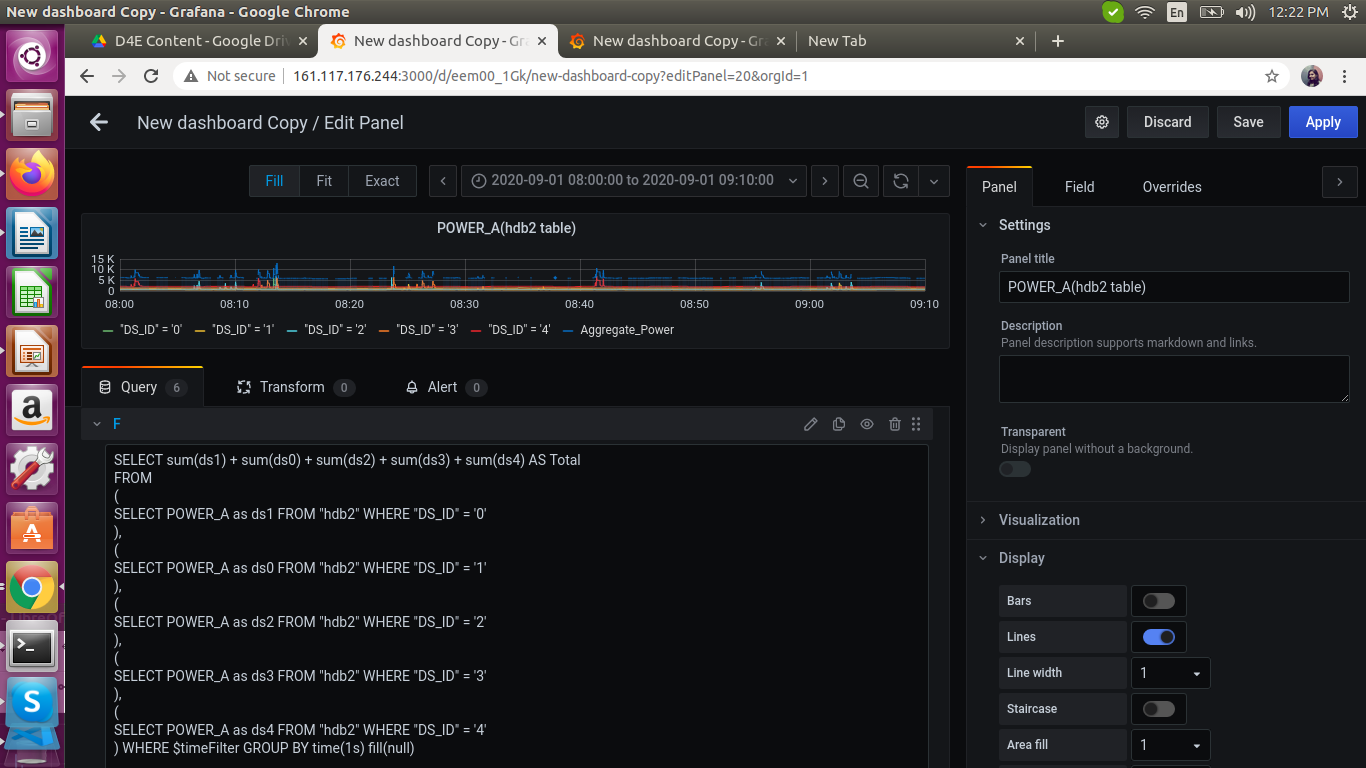We get the graph as shown below: TIMESTAMP SAMPLE POWER_A 2020-09-01 08:27:16 Sample 1 7.92k  TIMESTAMP SAMPLE POWER_A 2020-09-01 08:17:08 Sample 2 5.77k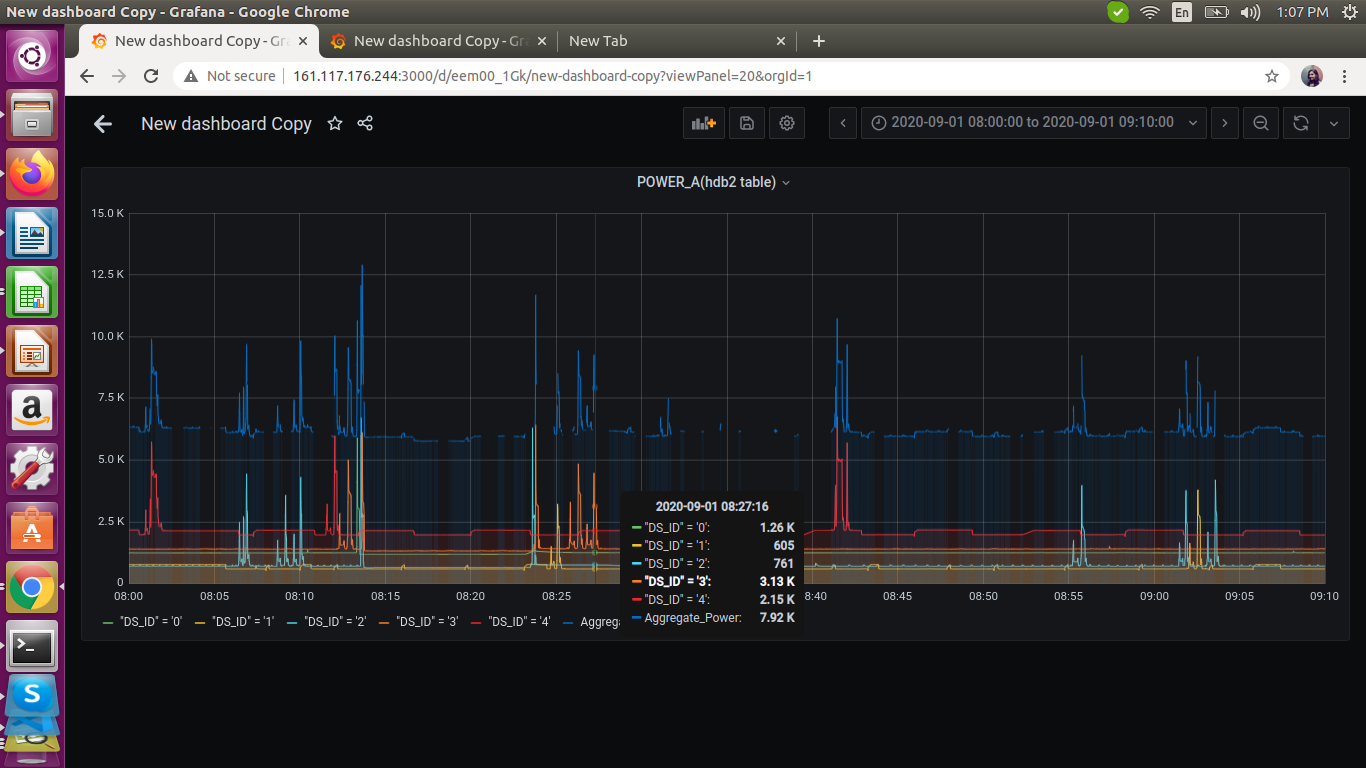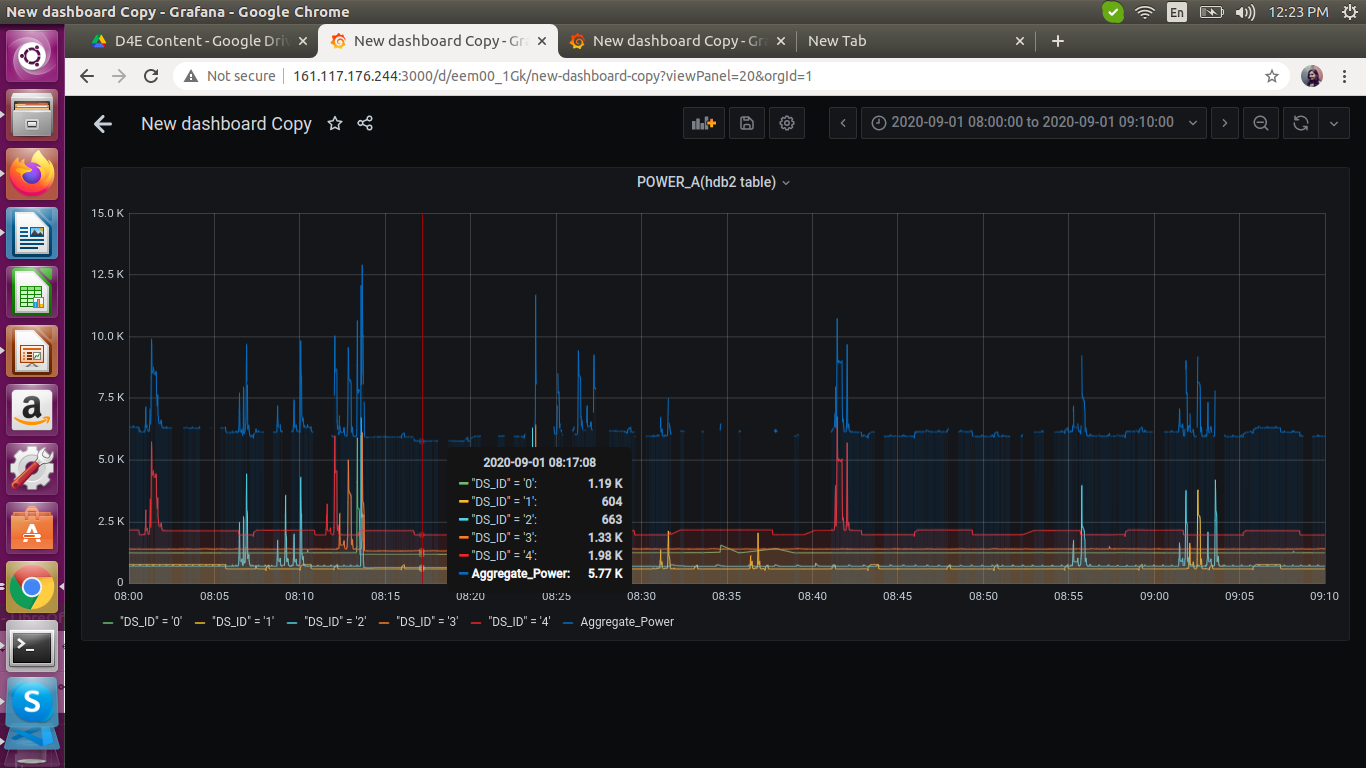We are getting the correct aggregated values for POWER_A. We need to do the same for POWER_B and POWER_C. For POWER_B: SELECT sum(ds1) + sum(ds0) + sum(ds2) + sum(ds3) + sum(ds4) AS PB FROM ( SELECT POWER_B as ds1 FROM "hdb2" WHERE "DS_ID" = '1' ), ( SELECT POWER_B as ds0 FROM "hdb2" WHERE "DS_ID" = '0' ), ( SELECT POWER_B as ds2 FROM "hdb2" WHERE "DS_ID" = '2' ), ( SELECT POWER_B as ds3 FROM "hdb2" WHERE "DS_ID" = '3' ), ( SELECT POWER_B as ds4 FROM "hdb2" WHERE "DS_ID" = '4' ) WHERE$timeFilter GROUP BY time(1s) fill(null)


For POWER_C:

SELECT sum(ds1) + sum(ds0) + sum(ds2) + sum(ds3) + sum(ds4) AS PC
FROM
(
SELECT POWER_C as ds1 FROM "hdb2" WHERE "DS_ID" = '1'
),
(
SELECT POWER_C as ds0 FROM "hdb2" WHERE "DS_ID" = '0'
),
(
SELECT POWER_C as ds2 FROM "hdb2" WHERE "DS_ID" = '2'
),
(
SELECT POWER_C as ds3 FROM "hdb2" WHERE "DS_ID" = '3'
),
(
SELECT POWER_C as ds4 FROM "hdb2" WHERE "DS_ID" = '4'
) WHERE $timeFilter GROUP BY time(1s) fill(null)  We will get the graph as shown below: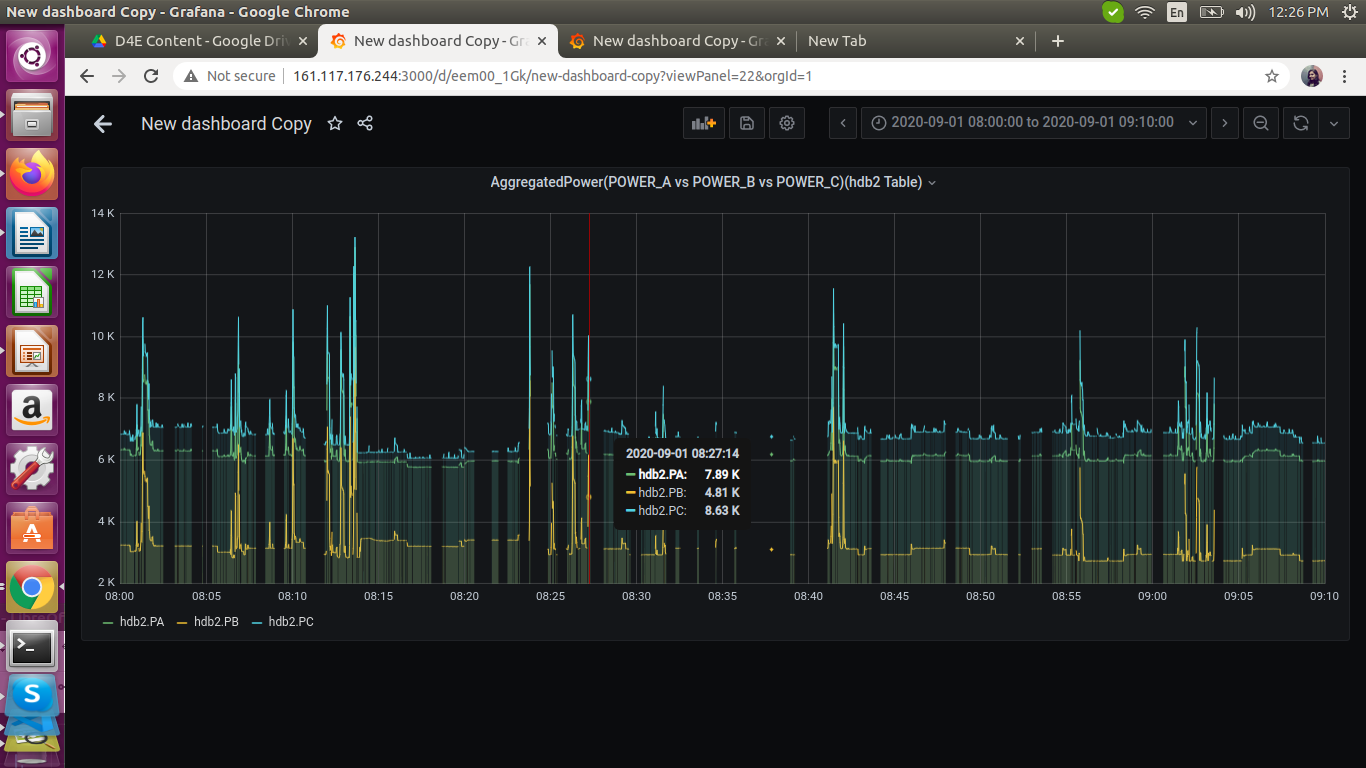|TIMESTAMP SAMPLE POWER_A 2020-09-01 08:27:12 Sample 3 7.89k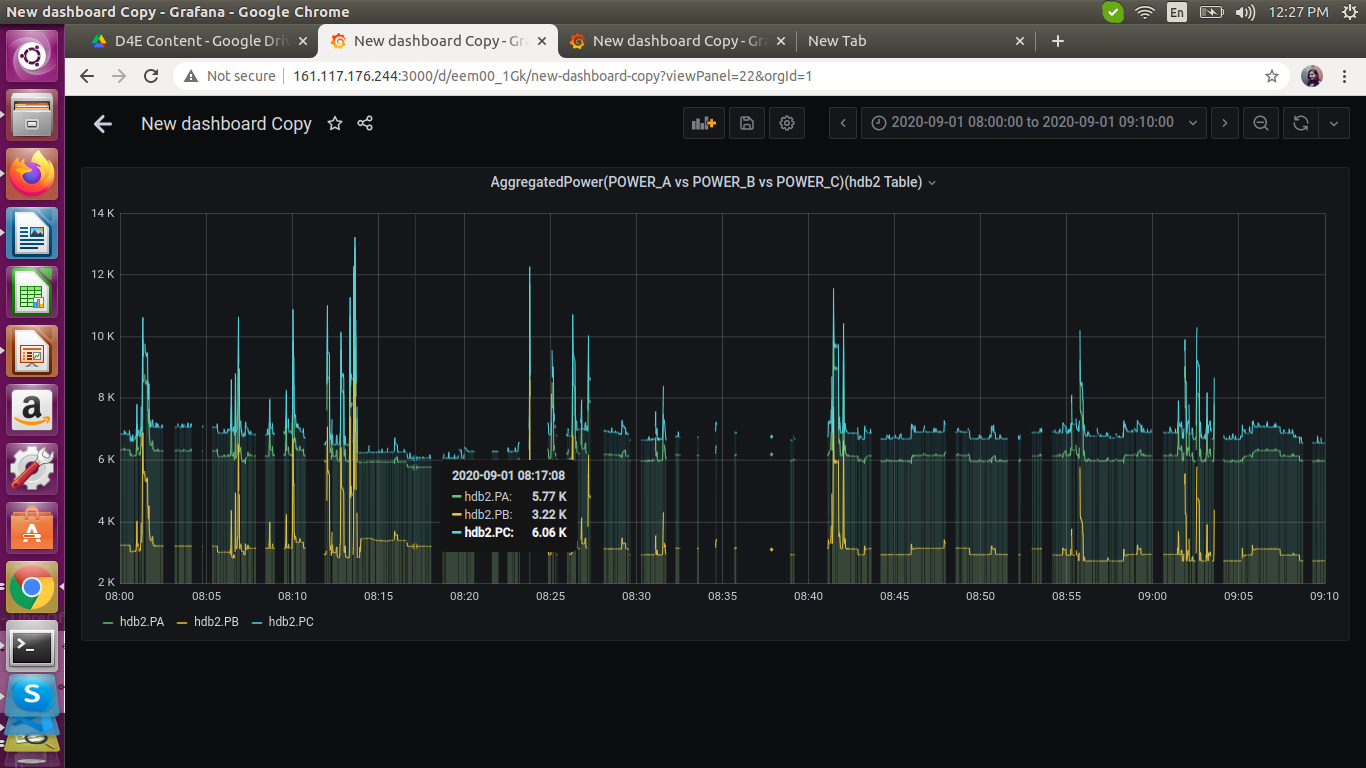TIMESTAMP SAMPLE POWER_A 2020-09-01 08:17:08 Sample 4 5.77k  ## PIE CHART VISUALIZATION: Below is the query for visualization of 3 components: Lift_Energy, Public_Lighting_Energy, Booster_Pump_Energy. SELECT (( (sum("LIFT_TOTAL")) / (($to - $from) / 1000) ) )* (($to - $from) / 3600000) as Lift_Energy, (((sum("LIGHT_TOTAL")) / (($to - $from) / 1000))) * ((($to - $from) / 1000) / 3600) as Public_Lighting_Energy, (((sum("PUMP_TOTAL")) / (($to - $from) / 1000))) * ((($to - $from) / 1000) / 3600) as Booster_Pump_Energy FROM "hdb2" WHERE$timeFilter GROUP BY time(24h)


Here, we have combined 3 subqueries.

(sum("LIFT_TOTAL")) / (($to -$from) / 1000) ) )* (($to -$from) / 3600000) as Lift_Energy

(sum("LIGHT_TOTAL")) / (($to -$from) / 1000))) * ((($to -$from) / 1000) / 3600) as Public_Lighting_Energy

(sum("PUMP_TOTAL")) / (($to -$from) / 1000))) * ((($to -$from) / 1000) / 3600) as Booster_Pump_Energy


All the 3 queries are similar. We will take 1st query. The records are taken for each second,i.e, for every consecutive second we are recording the data. Here, (sum("LIFT_TOTAL")) is the sum computed over the period mentioned - (($**to -$**from) in the time window.

SELECT
((    (sum("LIFT_TOTAL"))  / ((1599190800000 - 1598898600000) / 1000) ) )* ((1599190800000 - 1598898600000) /  3600000)
as Lift_Energy,
(((sum("LIGHT_TOTAL"))  / ((1599190800000 - 1598898600000) / 1000))) * (((1599190800000 - 1598898600000) / 1000) / 3600)
as Public_Lighting_Energy,
(((sum("PUMP_TOTAL"))  / ((1599190800000 - 1598898600000) / 1000))) * (((1599190800000 - 1598898600000) / 1000) / 3600) as Booster_Pump_Energy
FROM "hdb2" WHERE time >= 1598898600000ms and time <= 1599190800000ms GROUP BY time(24h)


Since the precision is in milliseconds, we are dividing it by 1000.Now, we get total power for the time range applied. The unit for energy consumption is watt-hour(Wh). Example, a 40-watt electric appliance operating continuously for 25 hours uses one kilowatt-hour. The value ((($__to - \_\_from\) / 1000\) / 3600\) gives the total operating hours which multiplied with the total power,i.e,$$sum\("PUMP\_TOTAL"$$\) / $$\(__to - __from) / 1000))) gives power consumption in watt-hour units.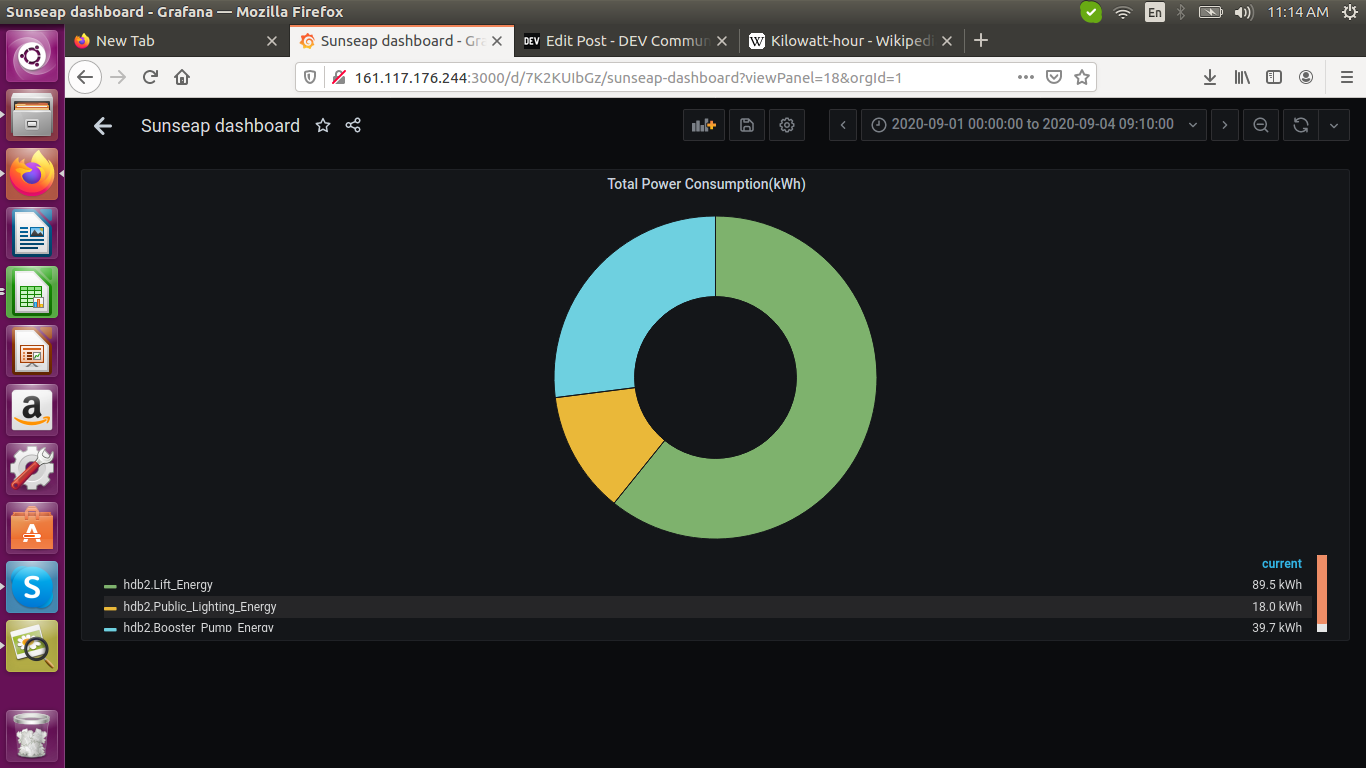WORLD MAP VISUALIZATION: The sample csv table we are using for the world map is shown below.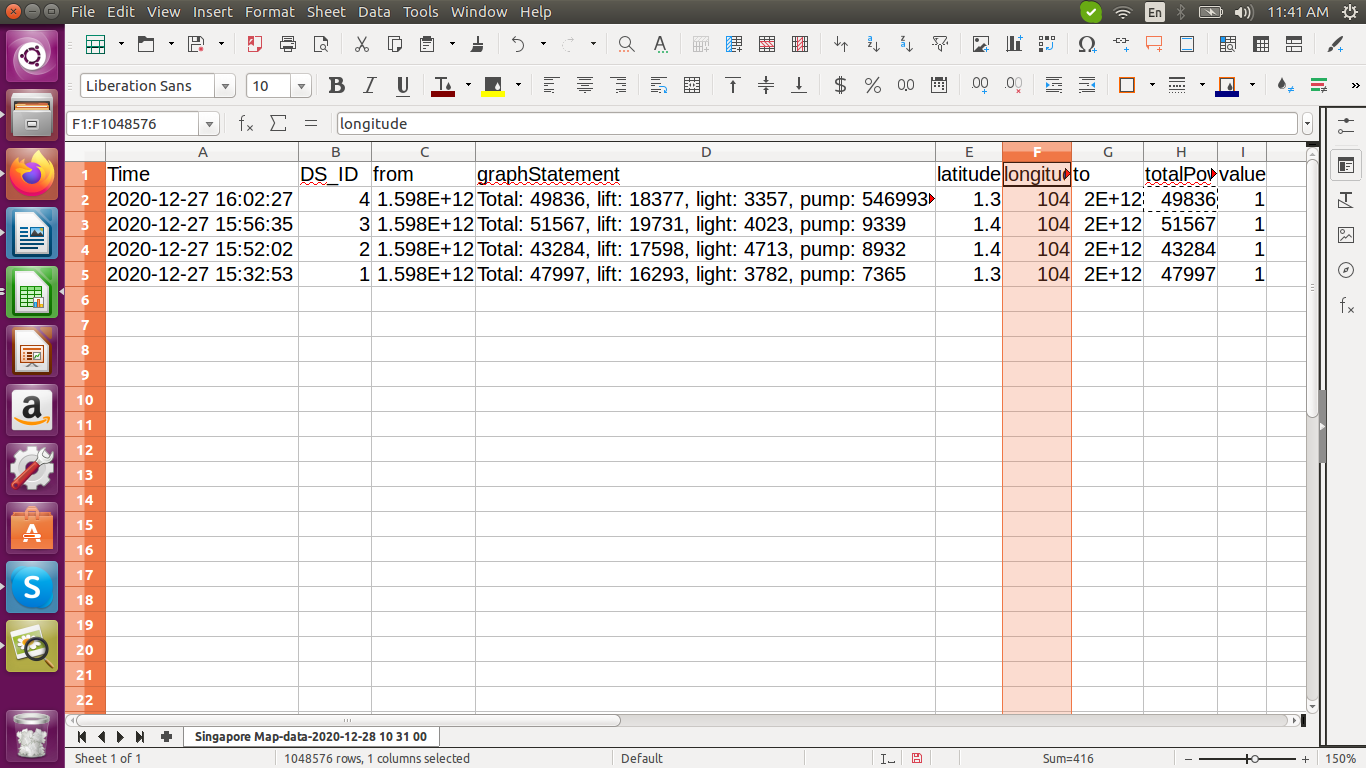Above is the csv file which we are uploading in the influxdb into the "hdb7" measurement. Query for world map: SELECT * from "hdb7" GROUP BY * ORDER BY DESC LIMIT 4  We can see the query and the settings also in the image below. Here, we are using latitude and longitude values to plot the map and put the graphStatement field in the label of the map.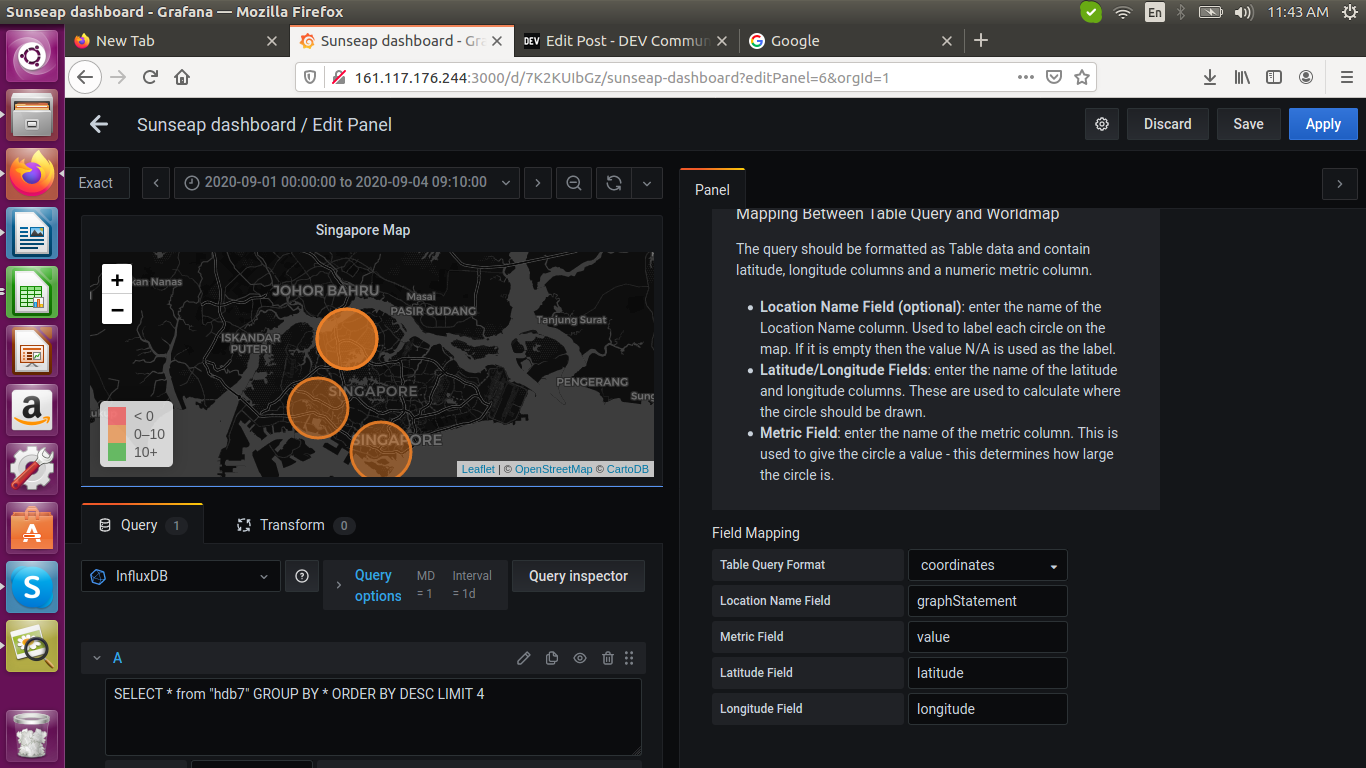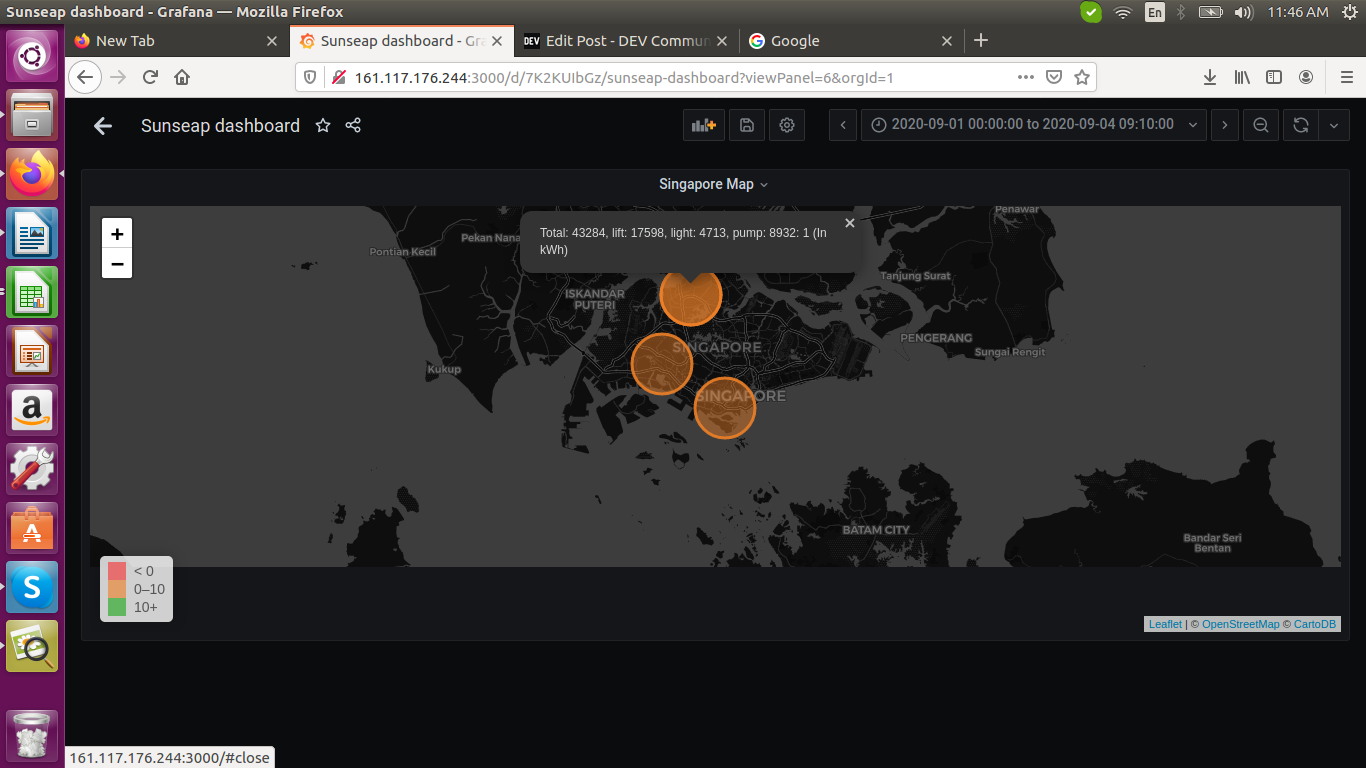b. More on Installing Plugins Docker Grafana: Open the SSH terminal on your machine and run the following command: ssh your_username@host_ip_address  If the username on your local machine matches the one on the server you are trying to connect to, you can just type: ssh host_ip_address  And hit Enter. After successful login, execute the below commands in the shell: sudo docker ps -a sudo docker exec -it --user=root grafana /bin/sh grafana-cli plugins install grafana-worldmap-panel sudo docker container stop d1ead747ec87 sudo docker start d1ead747ec87  1.The command  sudo docker ps -a  lists all the running containers. 2. We can execute/test the commands for the application running inside the container with sudo docker exec -it --user=root grafana /bin/sh  command. We can also ping to test the port connections with the commands: curl http://localhost:3000  curl http://160.100.100.204:3000  where the ip-address is of the remote virtual machine provisioned in the cloud which we have logged into. 3. The plugins are installed with grafana-cli plugins install grafana-worldmap-panel.  4. The docker conatiners are restarted with commands:  sudo docker container stop d1ead747ec87  sudo docker start d1ead747ec87   Last login: Fri Dec 18 19:37:21 2020 from 49.206.11.161 root@d4eViz:~# sudo docker ps -a CONTAINER ID IMAGE COMMAND CREATED STATUS PORTS NAMES d1ead747ec87 grafana/grafana "/run.sh" 46 hours ago Up 17 hours 0.0.0.0:3000->3000/tcp grafana root@d4eViz:~# sudo docker exec -it --user=root grafana /bin/sh /usr/share/grafana # grafana-cli plugins install grafana-worldmap-panel installing grafana-worldmap-panel @ 0.3.2 from: https://grafana.com/api/plugins/grafana-worldmap-panel/versions/0.3.2/download into: /var/lib/grafana/plugins ✔ Installed grafana-worldmap-panel successfully Restart grafana after installing plugins . /usr/share/grafana # sudo docker container stop d1ead747ec87 /bin/sh: sudo: not found /usr/share/grafana # root@d4eViz:~# root@d4eViz:~# sudo docker container stop d1ead747ec87 d1ead747ec87 root@d4eViz:~# sudo docker start d1ead747ec87 d1ead747ec87 root@d4eViz:~#  root@d4eViz:~# sudo docker rm -fv (sudo docker ps -aq) 08d6b4e38932 root@d4eViz:~# sudo docker run -d -p 3000:3000 --name=grafana -v grafana-storage:/var/lib/grafana grafana/grafana d1ead747ec87a566c5f8de5c36a705d3b8e1860f7e7dc78b2ea5bf2ef0f574d8 root@d4eViz:~# sudo docker ps -a CONTAINER ID IMAGE COMMAND CREATED STATUS PORTS NAMES d1ead747ec87 grafana/grafana "/run.sh" 4 seconds ago Up 4 seconds 0.0.0.0:3000->3000/tcp grafana root@d4eViz:~# sudo docker exec -it grafana /bin/sh /usr/share/grafana curl http://localhost:3000 /bin/sh: curl: not found /usr/share/grafana apk add curl ERROR: Unable to lock database: Permission denied ERROR: Failed to open apk database: Permission denied /usr/share/grafana root@d4eViz:~# root@d4eViz:~# sudo docker exec -it --user=root grafana /bin/sh /usr/share/grafana # apk add curl fetch http://dl-cdn.alpinelinux.org/alpine/v3.12/main/x86_64/APKINDEX.tar.gz fetch http://dl-cdn.alpinelinux.org/alpine/v3.12/community/x86_64/APKINDEX.tar.gz (1/3) Installing nghttp2-libs (1.41.0-r0) (2/3) Installing libcurl (7.69.1-r3) (3/3) Installing curl (7.69.1-r3) Executing busybox-1.31.1-r19.trigger Executing glibc-bin-2.30-r0.trigger /usr/glibc-compat/sbin/ldconfig: /usr/glibc-compat/lib/ld-linux-x86-64.so.2 is not a symbolic link OK: 27 MiB in 37 packages /usr/share/grafana # curl http://localhost:3000 Found. /usr/share/grafana # curl http://160.100.100.204:3000 Found. /usr/share/grafana #  c. Writing to the InfluxDB2.0 Requirements: Get started with InfluxDB 2.0 The InfluxDB 2.0 time series platform is purpose-built to collect, store, process and visualize metrics and events. Get started with InfluxDB OSS v2.0 by downloading InfluxDB, installing the necessary executables, and running the initial setup process. If not installed, follow the link https://docs.influxdata.com/influxdb/v2.0/get-started/ Start InfluxDB by running the influxd daemon: k@k-Lenovo-G50-70:~ influxd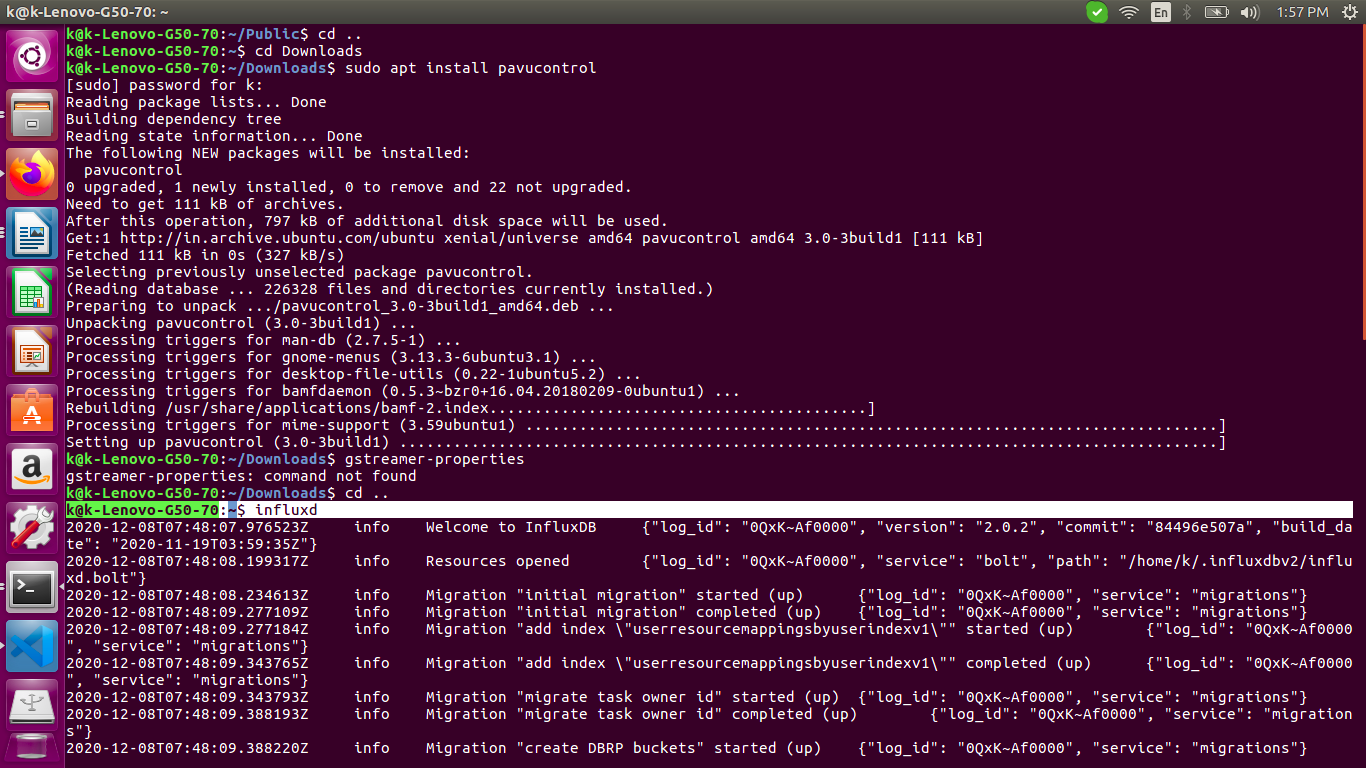Steps: 1. Replace the values of INFLUX_TOKEN, ORG, BUCKET_NAME and measurement_name with the name of the table you need to create. Also replace the csv path you need to upload the csv file at line: with open\('/home/k/Downloads/influxData/data\_0\_20200901.csv'$$ as csv\_file: In the csv file, we have time stored in Unix Timestamp format.  2. Run the below program: k@k-Lenovo-G50-70:~/a_b$ python3 pushToInflux2_0.py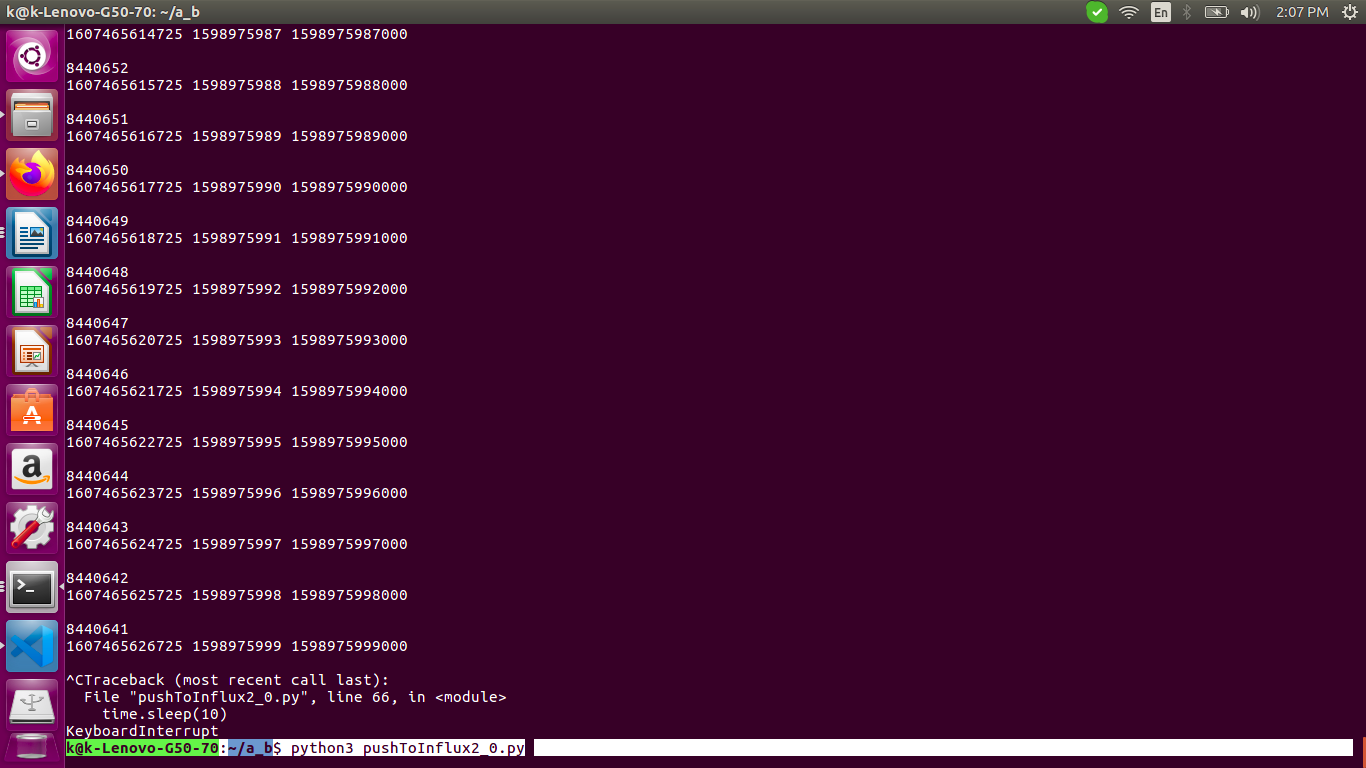'''
!/usr/bin/python
'''
import requests
import uuid
import random
import time
import sys
import csv
import json

INFLUX_TOKEN='qCAYOyvOErIP_KaJssk_neFar-o7PdvHL64eWYCD_ofywR_J3iubktdB58A3TE-6sM7C61Gt8qOUPvc4t0WVBg=='
ORG="asz"
INFLUX_CLOUD_URL='localhost'
BUCKET_NAME='b'
'''
Be sure to set precision to ms, not s
'''
QUERY_URI='http://{}:8086/api/v2/write?org={}&bucket={}&precision=ms'.format(INFLUX_CLOUD_URL,ORG,BUCKET_NAME)
measurement_name = 'data_0_20200901'
'''
Increase the points, 2, 10 etc.
'''
number_of_points = 1000
batch_size = 1000

print('Processed')
_row = 0
if row == "TIMESTAMP":
pass
else:
_row = int((int(row)) * 1000)
print(_data_end_time, row,_row, '\n')
data.append("{measurement},location={location} POWER_A={POWER_A},POWER_B={POWER_B},POWER_C={POWER_C} {timestamp}"
.format(measurement=measurement_name, location="reservoir", POWER_A=row, POWER_B=row, POWER_C=row, timestamp=_row))

count = 0
if name == 'main':
# Check to see if number of points factors into batch size
count = 0
if (  number_of_points % batch_size != 0 ):
raise SystemExit( 'Number of points must be divisible by batch size' )
# Newline delimit the data
for batch in range(0, len(data), batch_size):
time.sleep(10)
current_batch = '\n'.join( data[batch:batch + batch_size] )
print(current_batch)
count = count + 1
print(r.status_code, count, data[count])


In the InfluxDB2.0 screen page at: http://localhost:8086/orgs/772821f18f2f7a0d/data-explorer?bucket=b

Under the Explore optionWe need to set the WINDOW PERIOD as 15s / 1m to see more points on the dashboard.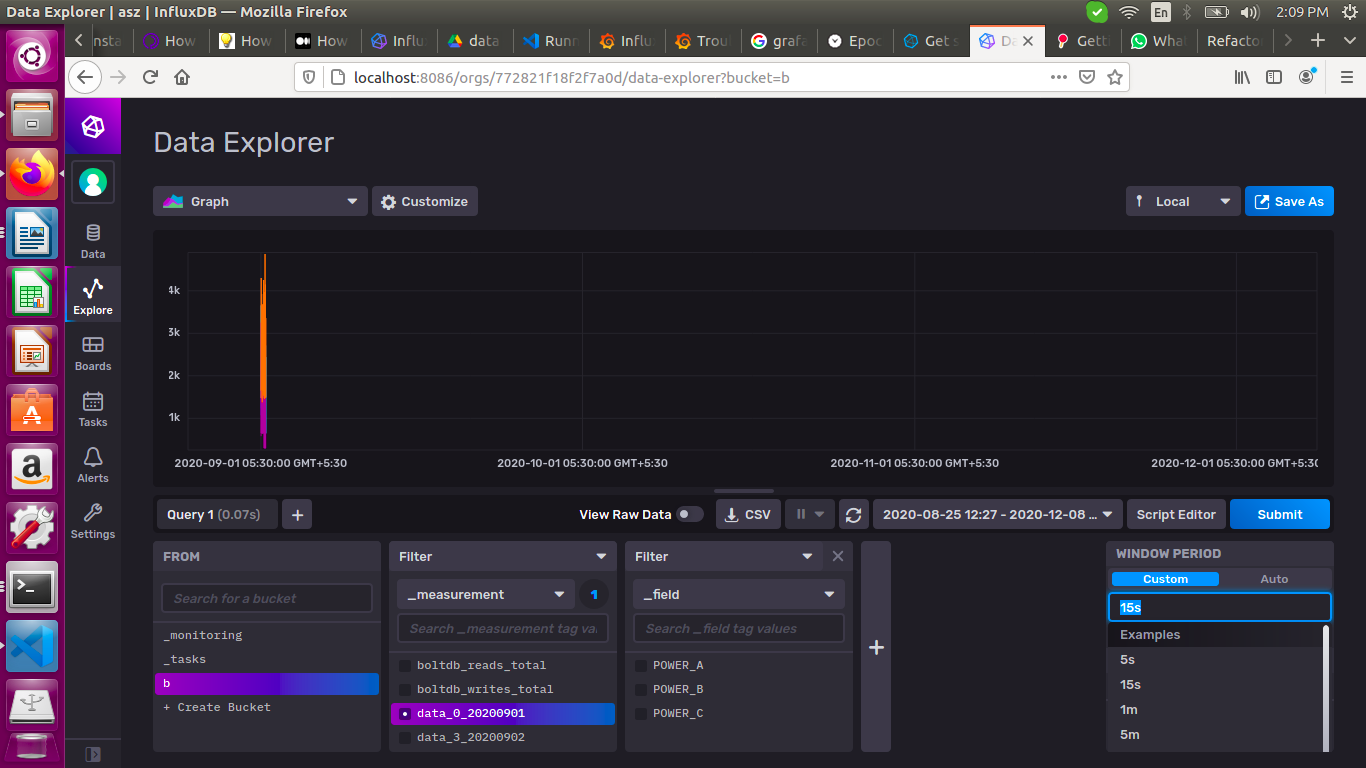Also, the timing window must be adjusted according to the timestamp of the record.

For example:

TIMESTAMP

DS_Id

POWER_A

POWER_B

POWER_C

1598918400

0

1403.421

712.372

1680.471

So, we need to apply the time range from the above date for the results to show as in the above window.

## My Github Profile for code:

Please see the master branch in my repo: https://github.com/krishnakurtakoti/python-influxdb-2.0-write

Also published at https://dev.to/krishnakurtakoti/grafana-dashboard-5f8Thanks!